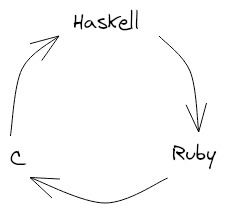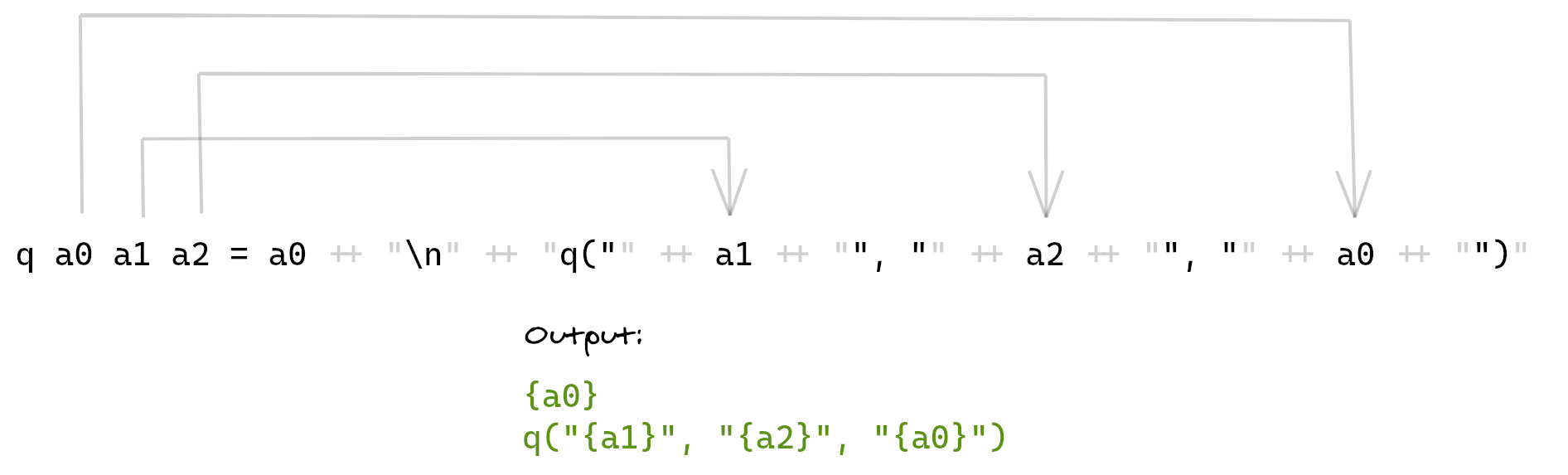# Understanding `quine-central`: how do quine loops work?

2020-09-24

Yesterday another Recurser introduced me to `quine-central`, which is:

[A] Haskell program that generates a Haskell program that prints out a Perl program that prints out a Python program that prints out a Ruby program that prints out a C program that prints out a Java program that prints out a Rust program that prints out an OCaml program that prints out a Swift program that prints out a Racket program that prints out a Javascript program that prints out the first Haskell program generated.

“How the hell does that work?” I thought to myself. Maybe you’re thinking that too, so here’s my attempt at an explanation.

`quine-central` can produce quine loops of any length, so let’s first think about a loop of three programming languages: Haskell, Ruby, and C. In this case, `quine-central` prints a Haskell program that prints a Ruby program that prints a C program that prints the first Haskell program generated.The generated Haskell program has two lines. The first line defines a function called `q` that takes three arguments. `q` builds and returns a string that contains its first argument, then a newline, and finally some Ruby code that calls a function called `q`. This call to `q` in Ruby takes the same arguments but rotated one position to the left. That is, it takes the second argument to the Haskell `q`, then the third, then the first.In the actual output, `{a0}` is replaced with the value of `a0`. Same goes for the other variables. Also, this isn’t quite the actual code. I simplified it a little for demonstration purposes.

The generated Haskell program's second line is a main method that calls the function `q` defined on the first line and prints the result. Each argument passed to `q` is the source code for a definition of `q` in a language in the loop. The first argument is a definition of `q` in Ruby, the second in C, the last in Haskell.

``````main = putStrLn \$ q "{Ruby    definition of q}"
"{C       definition of q}"
In all of these definitions, `q` does the same thing as it does in the Haskell program. It prints its first argument, then prints a call to a function called `q`, but in the next language in the loop. It also rotates its arguments one position to the left before printing them.
When the program is run, `q` starts by printing its first argument, which is a declaration of `q` in Ruby. Then, it prints a call to `q` in Ruby. The arguments to this call are the second, third, and first arguments passed to `q` in the Haskell program, which are definitions of `q` in C, Haskell, and Ruby respectively.
The output of the Haskell program is a Ruby program with a very similar structure. Both have two lines. Both declare a function `q` on the first line. `q` does the same thing in both programs. And both call `q` on the second line, passing in definitions of `q` for the rest of the loop.
In the next post, I’ll discuss how `quine-central` generates these programs in the first place. We’ll also do away with some of the simplifications I made to the programs in this post and look at how `quine-central` handles the problems posed by string escaping.